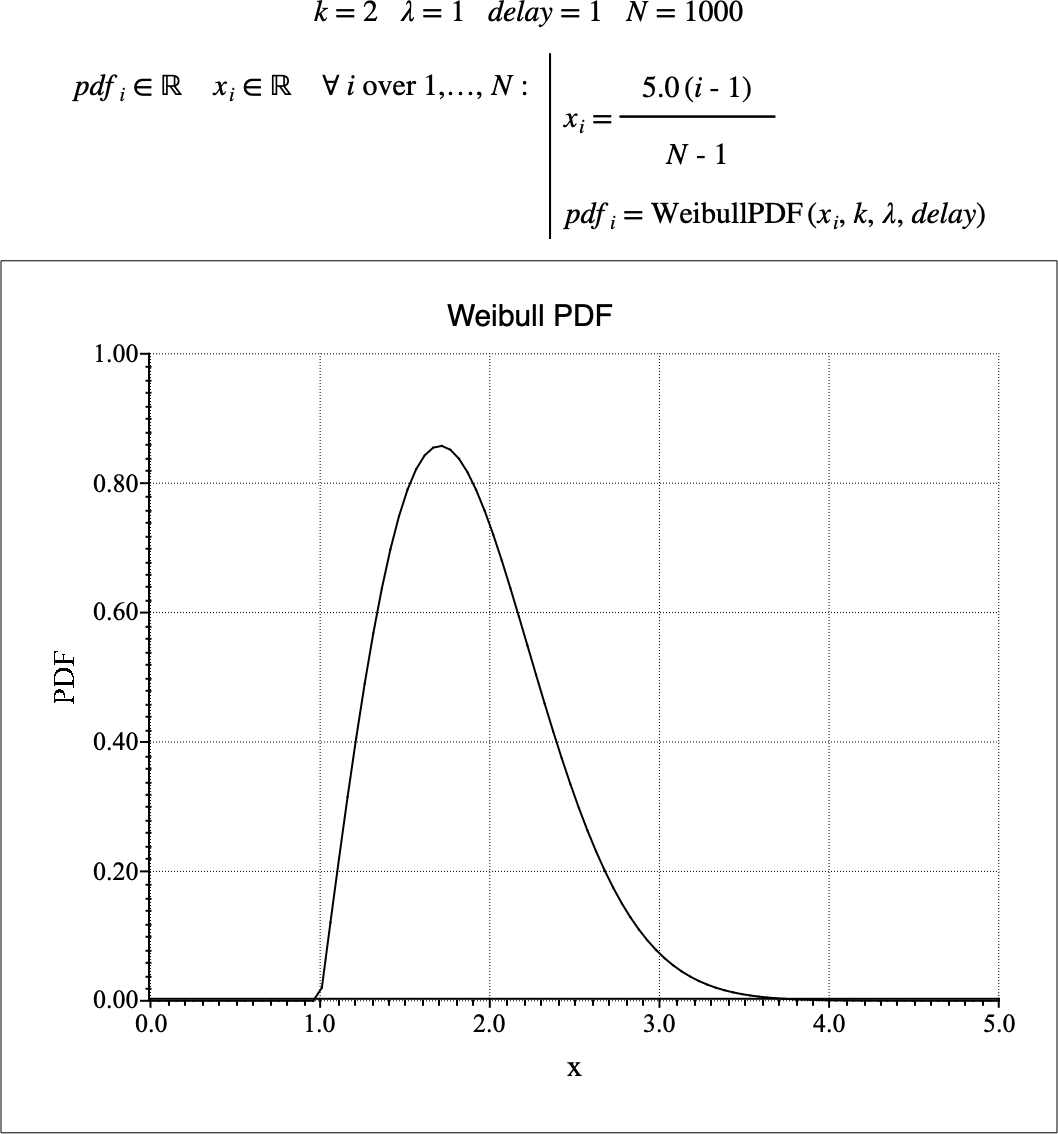# $$\text{WeibullPDF}$$¶

You can use the $$\text{WeibullPDF}$$ function to calculate the probability density function (PDF) of the Weibull distribution.

You can use the \weibullp backslash command to insert this function.

The following variants of this function are available:

• $$\text{real } \text{WeibullPDF} \left ( \text{<x>}, \text{<k>}, \text{<}\lambda\text{>} \right )$$

• $$\text{real } \text{WeibullPDF} \left ( \text{<x>}, \text{<k>}, \text{<}\lambda\text{>}, \text{<delay>} \right )$$

Where $$\text{<x>}$$, $$\text{<k>}$$, and $$\text{<}\lambda\text{>}$$ are scalar values representing the value of interest, the shape term, and the scale term respectively. Th $$\text{WeibullPDF}$$ function is defined over the range $$k > 0$$, and $$\lambda > 0$$ and will generate an error or return NaN for values for which the function is not defined.

The $$\text{<delay>}$$ term is optional. If not specified, a delay of 0 is used.

The value is calculated directly using the relation:

$\begin{split}\text{WeibullPDF} \left ( x, k, \lambda, delay \right ) = \begin{cases} \frac{k}{\lambda} \left ( \frac{x - delay}{\lambda} \right ) ^ { k - 1 } e ^ { - \left( \frac{x - delay}{\lambda} \right ) ^ k } & \text{if } x \geq delay \\ 0 & \text{otherwise} \end{cases}\end{split}$

Figure 217 shows the basic use of the $$\text{WeibullPDF}$$ function.Figure 217 Example Use Of the WeibullPDF Function